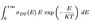# I want to know how I can plot Nuclear reaction rates when the equation depends on the fusion cross section of the reaction

Rhony
TL;DR Summary
I know how to get fusion cross section plots from the exfor website but now I need to plot the reaction rate as a function of temperature. According to the image, I have sigma and E values as x and y data. How do I integrate using matlab .
I know how to get fusion cross section plots from the exfor website but now I need to plot the reaction rate as a function of temperature. According to the image, I have sigma and E values as x and y data. How do I integrate using MATLAB .

#### Attachments

•Capture.PNG
1.2 KB · Views: 99

Staff Emeritus
It would seem this is a MATLAB numerical integration problem.

So one has a nuclear (nuclei) energy spectrum, and I assume T is the average temperature (related to <E>) of the nuclei in the plasma. Is this correct?

Rhony
Yes that's correct. I had got pb11 cross section data points from exfor. Now using maxwellian conditions, I wanted to use those to plot reaction rate. Its done in many textbooks where the explain the difference between cross section and reaction rate, but they never mention the steps followed.

rpp
Do you have a plot of the cross sections, or a table of the cross section values? You will need the table of values.
If you only have the plot, check the website again to see if there is a way to download the raw data.

Next, check to see if the cross sections are evaluated at "E", are are they differential cross sections as a function of E? (sigma(E)). I've seen it both ways.
If they are not differential cross sections, you will need to divide the cross section by the appropriate "delta-E" to put it in differential form.

Now you have:
• Values of sigma(E)
• T is a constant value
• You can evaluate E*exp()
It is just a matter of performing the numerical integration.

rpp
Do you have a plot of the cross sections, or a table of the cross section values? You will need the table of values.
If you only have the plot, check the website again to see if there is a way to download the raw data.

Next, check to see if the cross sections are evaluated at "E", are are they differential cross sections as a function of E? (sigma(E)). I've seen it both ways.
If they are not differential cross sections, you will need to divide the cross section by the appropriate "delta-E" to put it in differential form.

Now you have:
• Values of sigma(E)
• T is a constant value
• You can evaluate E*exp()
It is just a matter of performing the numerical integration.
I just realized my last post was incorrect, and I don't see a way to edit it. The cross sections are always going to be evaluated at "E", you should never have to divide by "delta-E". It is the flux that can be a function of E. Sorry for the confusion.

Mentors: is there a way to edit my previous post?